﻿ 轴向外伸欧拉梁减振时域数值仿真 Numerical Simulation in Time Domain of Vibration Reduction of Axially Extended Euler Beam

International Journal of Mechanics Research
Vol.06 No.04(2017), Article ID:23027,7 pages
10.12677/IJM.2017.64018

Numerical Simulation in Time Domain of Vibration Reduction of Axially Extended Euler Beam

Teng He, Shuo Wang, Bo Fang

Faculty of Aerospace Engineering, Shenyang Aerospace University, Shenyang Liaoning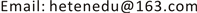Received: Nov. 23rd, 2017; accepted: Dec. 7th, 2017; published: Dec. 14th, 2017ABSTRACT

In this paper, using the Hamilton principle, the nonlinear dynamics equation of an axially moving homogenous and isotropic beam with the additional nonlinear energy sink is derived. The beam, length changes with time, is based on the Euler Bernoulli beam model. Then, transforming the equations to dimensionless ones, using the Galerkin method, the governing equations are truncated. Meanwhile, the nonlinear ordinary differential equation, reflecting the transverse vibration of the telescopic cantilever beam, is obtained. The vibration characteristics of the model in the process of overhang and recovery are analyzed in time domain by numerical calculation method after appropriate nonlinear energy sink parameters were designed. The results show that the nonlinear energy sink has good effect on vibration suppression.

Keywords:Extensible Beam, Isotropic Cantilever Beam, Nonlinear Energy Sink, Nonlinear Dynamics1. 引言

2. 外伸欧拉梁动力学方程

${\epsilon }_{x}=-z\frac{{\partial }^{2}\omega }{\partial {x}^{2}}$ (1)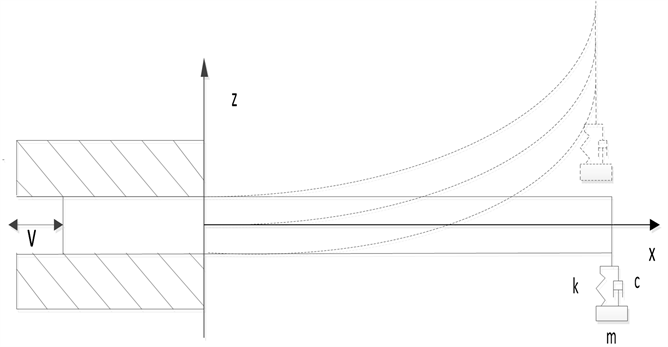Figure 1. Model diagram

$\sigma =E{\epsilon }_{x}$ (2)

$U=\frac{1}{2}{\int }_{V}\sigma \epsilon {\text{d}}_{V}$ (3)

$T={\int }_{v}\left[\rho {\left(\frac{\partial \omega }{\partial t}+{V}_{0}\frac{\partial \omega }{\partial x}\right)}^{2}+\rho {V}_{0}^{2}\right]\text{d}v$ (4)

$W={\int }_{0}^{l\left(t\right)}\left[k{\left(y-\omega \right)}^{3}+c\left(\frac{\text{d}y}{\text{d}t}-\frac{\text{d}\omega }{\text{d}t}\right)\right]\delta \left(y-d\right)\delta \omega \text{d}x$ (5)

${\int }_{{t}_{0}}^{{t}_{1}}\left(\delta T+\delta U-\delta W\right)\text{d}t=0$ (6)

$\rho bh\left(\frac{{\partial }^{2}\omega }{\partial {t}^{2}}+2{V}_{0}\frac{{\partial }^{2}\omega }{\partial x\partial t}+{V}_{0}{}^{2}\frac{{\partial }^{2}\omega }{\partial {x}^{2}}\right)+EI\frac{{\partial }^{4}\omega }{\partial {x}^{4}}=\left[k{\left(y-\omega \right)}^{3}+c\left(\frac{\text{d}y}{\text{d}t}-\frac{\text{d}\omega }{\text{d}t}\right)\right]\delta \left(x-d\right)$ (7-1)

$m\frac{{\text{d}}^{2}y}{\text{d}{t}^{2}}+k{\left(y-\omega \right)}^{3}+c\left(\frac{\text{d}y}{\text{d}t}-\frac{\text{d}\omega }{\text{d}t}\right)=0$ (7-2)

$\begin{array}{l}\overline{x}=\frac{x}{{l}_{0}},\text{\hspace{0.17em}}\overline{l}=\frac{l}{{l}_{0}},\text{\hspace{0.17em}}\overline{\eta }=\frac{\omega }{h},\text{\hspace{0.17em}}y=\frac{y}{h},\text{\hspace{0.17em}}\overline{t}=\tau t,\text{\hspace{0.17em}}\tau =\frac{1}{{l}_{0}{}^{2}}\sqrt{\frac{EI}{\rho bh}},\\ \overline{d}=\frac{d}{{l}_{0}},\text{\hspace{0.17em}}{A}_{1}=\frac{k{l}_{0}{}^{3}{h}^{2}}{EI},\text{\hspace{0.17em}}{A}_{2}=\frac{C}{\rho bh\tau {l}_{0}},\text{\hspace{0.17em}}\epsilon =\frac{m}{\rho bh}\end{array}$

$\frac{{\partial }^{2}\overline{\eta }}{\partial {\overline{t}}^{2}}+2\frac{\text{d}\overline{x}}{\text{d}\overline{t}}\frac{{\partial }^{2}\overline{\eta }}{\partial \overline{x}\partial \overline{t}}+{\left(\frac{\text{d}\overline{x}}{\text{d}\overline{t}}\right)}^{2}\frac{{\partial }^{2}\overline{\eta }}{\partial {\overline{x}}^{2}}+\frac{{\partial }^{4}\overline{\eta }}{\partial {\overline{x}}^{4}}={A}_{1}{\left(\overline{y}-\overline{\eta }\right)}^{3}+{A}_{2}\left(\frac{\text{d}\overline{y}}{\text{d}\overline{t}}-\frac{\text{d}\overline{\eta }}{\text{d}\overline{t}}\right)$ (8-1)

$\epsilon \frac{{\text{d}}^{2}\overline{y}}{\text{d}{\overline{t}}^{2}}+{A}_{1}{\left(\overline{y}-\overline{\eta }\right)}^{3}+{A}_{2}\left(\frac{\text{d}\overline{y}}{\text{d}\overline{t}}-\frac{\text{d}\overline{\eta }}{\text{d}\overline{t}}\right)=0$ (8-2)

3. 伽辽金离散

${w}_{n}=\left[\mathrm{cosh}\left({\beta }_{n}x\right)-\mathrm{cos}\left({\beta }_{n}x\right)\right]-{\alpha }_{n}\left[\mathrm{sinh}\left({\beta }_{n}x\right)-\mathrm{sin}\left({\beta }_{n}x\right)\right]$ (9)

${\beta }_{n}$ 则由超越方程 $1+\mathrm{cosh}{\beta }_{n}\mathrm{cos}{\beta }_{n}=0$ 求出。于是可以设横向假设模态为：

${\eta }_{n}={\sum }_{n=1}^{n}{w}_{n}{\phi }_{n}\left(t\right)$ (10)

$\begin{array}{l}{M}_{22}\frac{{\text{d}}^{2}\phi }{\text{d}{t}^{2}}+{C}_{22}\frac{\text{d}\phi }{\text{d}t}+{K}_{22}\phi \\ =\left[{A}_{1}{\left(\overline{y}-{w}_{n}\left(x=1\right){\phi }_{n}\left(t\right)\right)}^{3}+{A}_{2}\left(\frac{\text{d}\overline{y}}{\text{d}\overline{t}}-{w}_{n}\left(x=1\right)\frac{\text{d}{\phi }_{n}\left(t\right)}{\text{d}\overline{t}}\right)\right]{w}_{n}\left(x=1\right)\\ \epsilon \frac{{\text{d}}^{2}\overline{y}}{\text{d}{\overline{t}}^{2}}+{A}_{1}{\left(\overline{y}-{w}_{n}\left(x=1\right){\phi }_{n}\left(t\right)\right)}^{3}+{A}_{2}\left(\frac{\text{d}\overline{y}}{\text{d}\overline{t}}-{w}_{n}\left(x=1\right)\frac{\text{d}{\phi }_{n}\left(t\right)}{\text{d}\overline{t}}\right)=0\end{array}$ (11)

4. 时域数值仿真

${\phi }_{1}=0,\text{\hspace{0.17em}}\text{\hspace{0.17em}}{\stackrel{˙}{\phi }}_{1}=0.1,\text{\hspace{0.17em}}\text{\hspace{0.17em}}{\phi }_{2}=0,\text{\hspace{0.17em}}\text{\hspace{0.17em}}{\stackrel{˙}{\phi }}_{2}=0$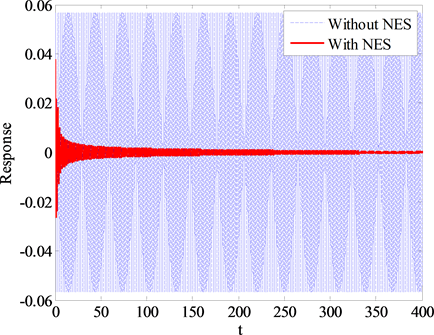Figure 2. $\overline{l}=1\text{\hspace{0.17em}}\text{m}$ oscillogram of the beam end(a)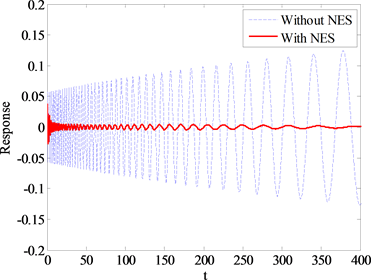(b)

Figure 3. (a) $\overline{l}=\left(1+0.01t\right)\text{\hspace{0.17em}}\text{m}$ oscillogram of the beam end; (b) $\overline{l}=\left(1-0.01t\right)\text{\hspace{0.17em}}\text{m}$ oscillogram of the beam end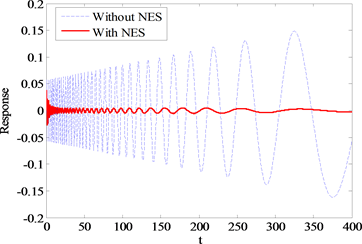(a)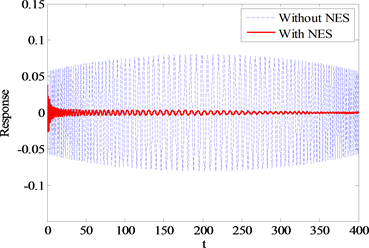(b)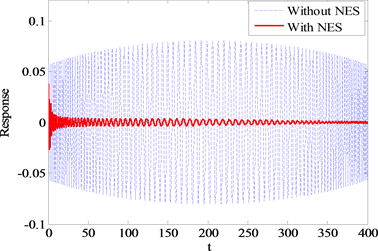(c)

Figure 4. (a) $\overline{l}=\left(1+0.01t-0.0005{t}^{2}\right)\text{m}$ oscillogram of the beam end; (b) $\overline{l}=1\text{\hspace{0.17em}}\text{m}$ and ${V}_{0}=0.01+0.001\mathrm{cos}t$ oscillogram of the beam end; (c) $\overline{l}=1\text{\hspace{0.17em}}\text{m}$ and ${V}_{0}=0.01-0.001\mathrm{cos}t$ oscillogram of the beam end

5. 结论

1) 在梁外伸过程中，末端的振幅随时间越来越大；在梁回收过程中，末端的振幅随时间越来越小；在外伸过程中梁伸缩到一定长度会发生失稳现象，此时结构力学性能被破坏。

2) 非线性能量阱能有效的抑制梁末端的振动情况。在梁外伸过程中，初始阶段即可有效的抑制振动，并且有效的抑制梁末端振幅随时间的增大；在梁回收过程中，NES可以减小梁末端的振幅。

Numerical Simulation in Time Domain of Vibration Reduction of Axially Extended Euler Beam[J]. 力学研究, 2017, 06(04): 173-179. http://dx.doi.org/10.12677/IJM.2017.64018

1. 1. Tabarrok, B., Leech, C.M. and Kim, Y.I. (1974) On the Dynamics of an Axially Moving Beam. Journal of the Franklin Institute, 297, 201-220. https://doi.org/10.1016/0016-0032(74)90104-5

2. 2. 李山虎, 杨靖波, 黄清华, 等. 轴向运动悬臂梁的独立模态振动控制-I近似理论分析[J]. 应用力学学报, 2002, 19(1): 35-39.

3. 3. 王亮, 陈怀海, 贺旭东, 等. 轴向运动变长度悬臂梁的振动控制[J]. 振动工程学报, 2009, 22(6): 565-570.

4. 4. Wang, L.H., Hu, Z.D., Zhong, Z. and Ju, J.W. (2009) Hamiltonian Dynamic Analysis of an Axially Translating Beam Featuring Time-Variant Velocity. Acta Mechanica, 206, 149-161. https://doi.org/10.1007/s00707-008-0104-9

5. 5. Bichiou, Y., Hajj, M.R. and Nayfeh, A.H. (2016) Effectiveness of a Nonlinear Energy Sink in the Control of an Aeroelastic System. Nonlinear Dynastic, 86, 2161-2177.

6. 6. Zhang, Y.W., Wang, C., Yuan, B., et al. (2017) Integration of Geometrical and Material Nonlinear Energy Sink with Piezoelectric Material Energy Harvester. Shock and Vibration, 2017, Article ID: 1987456. https://doi.org/10.1155/2017/1987456

7. 7. 刘天维, 林益明, 王明宇, 等. 航天器振动控制技术进展[J]. 宇航学报, 2008, 29(1): 1-12.

8. 8. 张也弛. 非线性能量阱的力学特性与振动抑制效果研究[D]: [博士学位论文]. 哈尔滨: 哈尔滨工业大学, 2012.

9. 9. 朱玉龙, 方勃. 考虑剪切变形的复合材料伸缩梁的动力学建模[J]. 工业, 2016, 6(1): 286.

10. 10. 朱玉龙. 基于NES的航天器伸缩结构的振动抑制[D]: [硕士学位论文]. 沈阳: 沈阳航空航天大学, 2016.